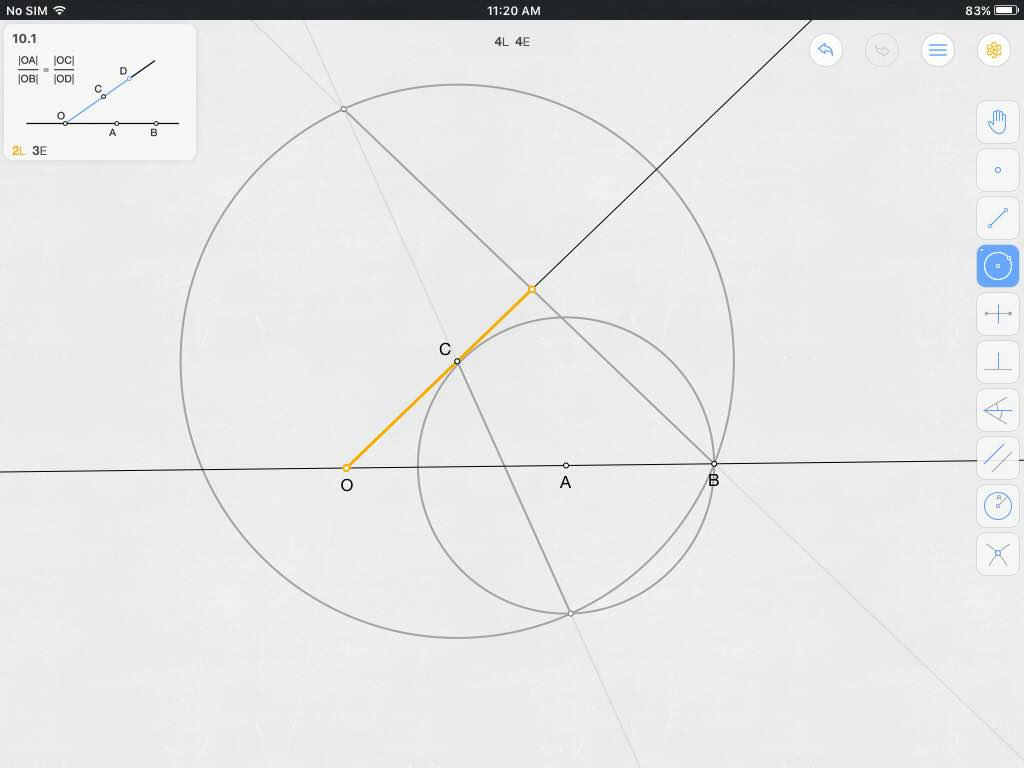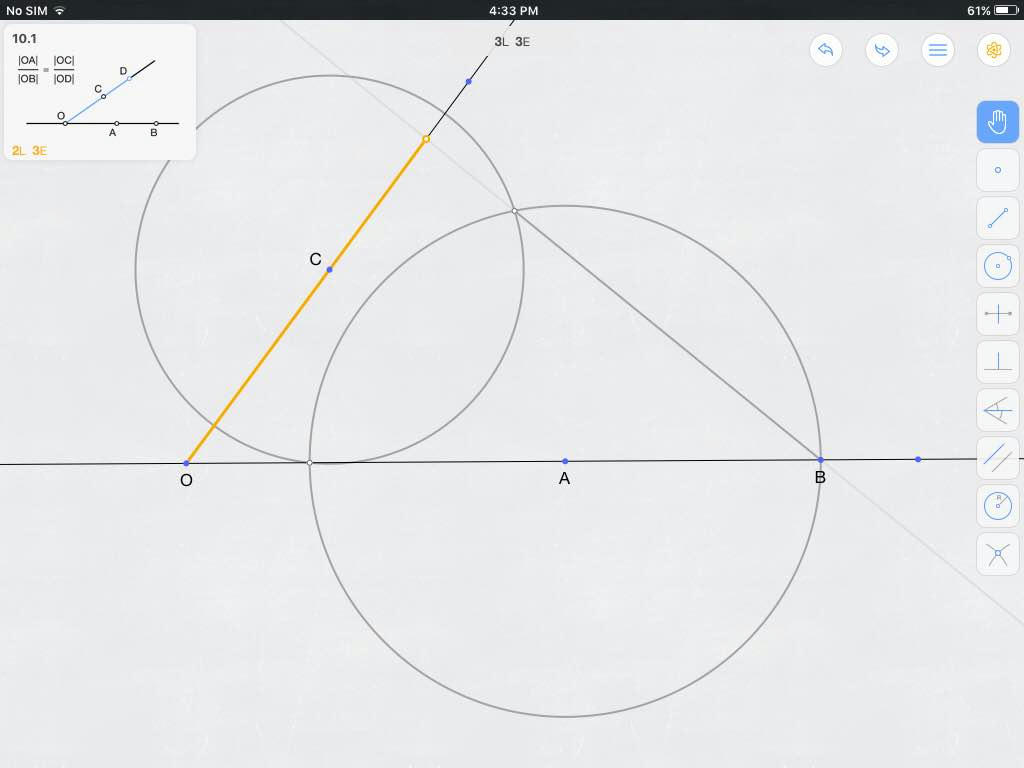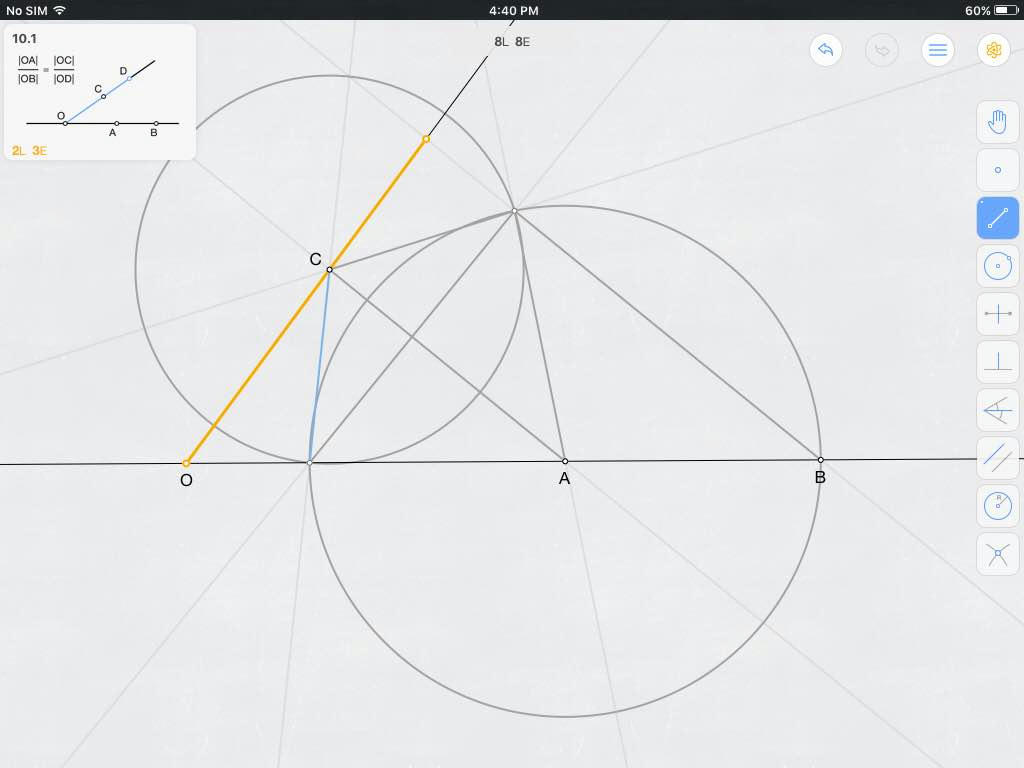# Three-step construction: Euclid's fourth proportional

PROBLEM Straight from Euclid's proposition on the fourth proportional, here is a surprisingly intriguing historical challenge posed on Euclidea, a mobile app for Euclidean constructions. I have a geometric construction that achieves the goal but not within the constraints. Could anyone advise on a construction that would fit within these constraints?

Given $\angle{O}$. Points $A$ and $B$ are on one ray of $\angle{O}$ in the order $O-A-B$, and $\overleftrightarrow{OA}$ is completely preconstructed. Point $C$ is on the other ray of $\angle{O}$.

Goal Construct point $D$ on the same ray of $\angle{O}$ as $C$, such that $$\frac{OA}{OB} = \frac{OC}{OD}.$$

Constraints Only an unmarked straightedge and a non-rusty collapsible compass (i.e., a compass that, while on paper, can have any radius but which cannot maintain said radius when not on paper) can be used. Use only from the construction steps below a number of steps that together require just three elementary steps in total.

1. Construct a point: 0 steps (S).
2. Mark the intersection of two curves with a point: 0S.
3. Construct a new line (*or line segment or ray): 1S.
4. Extend a given line segment (*or ray): 1S.
5. Construct a circle (non-rusty collapsible compass): 1S.
6. Construct the perpendicular bisector of a line segment: 3S.
7. Construct a new line perpendicular to an old line: 3S.

Hints from Euclidea A known solution employs three constructions in the order: Circle, circle, line.

PREVIOUS WORK Following Euclid himself, I constructed below a line $\overleftrightarrow{BF} \parallel \overline{OA}$; $\overleftrightarrow{BF}$ intersects $\overleftrightarrow{OC}$ at the required point $D$. But my construction barely misses both the 3S-constraint and the hint. The following figure accompanies my four-step construction, where the given and goal are shown as an inset:1. Construct $\bigcirc{A(B)}$ centered on point $A$ and with radius $AB$ [1S running total].
2. Construct $\bigcirc{C(B)}$ centered on point $C$ [2S running total].
• $\bigcirc{A(B)}$ also intersects $\bigcirc{C(B)}$ at another point $E \ne B$.
3. Construct $\overleftrightarrow{CE}$ [3S running total].
• $\overleftrightarrow{CE}$ also intersects $\bigcirc{C(B)}$ at another point $F \ne E$.
• Note that $\overline{EF}$ is a diameter of $\bigcirc{C(B)}$.
4. Construct $\overleftrightarrow{BF}$ [4S running total].
• $\overleftrightarrow{BF}$ intersects $\overleftrightarrow{OC}$ at the required point $D$. $\blacksquare$

## 1 Answer

SOLUTION Stumbled upon this construction that meets the constraint of using only three elementary steps. As noted for the previous construction, Euclid himself notes that the required point $D$ is the intersection of $\overleftrightarrow{OC}$ on one hand and of a line $\overleftrightarrow{BF} \parallel \overline{OA}$.1. Construct $\bigcirc{A(B)}$ centered on point $A$ and with radius $AB$ [1S running total].
• $\bigcirc{A(B)}$ also intersects $\overleftrightarrow{OB}$ at another point $E \ne B$.
2. Construct $\bigcirc{C(E)}$ [2S running total].
• $\bigcirc{C(E)}$ also intersects $\bigcirc{A(B)}$ at another point $F \ne E$.
3. Construct $\overleftrightarrow{BF}$ [3S running total].
• $\overleftrightarrow{BF}$ intersects $\overleftrightarrow{OC}$ at the required point $D$.

PROOF OF SOLUTION Auxiliary lines were added to the construction figure above, resulting in the proof figure below. Points common to both figures are named as they are in the construction figure.1. $\overline{AC} \cong \overline{AC}$ by identity, $\overline{AE} \cong \overline{AF}$ as radii of $\bigcirc{A(E)}$, and $\overline{CE} \cong \overline{CF}$ as radii of $\bigcirc{C(E)}$. Therefore, $\Delta{CAE} \cong \Delta{CAF}$ by SSS congruence, and thus $\angle{CAE} \cong \angle{CAF}$.
2. Let $G$ be the intersection of $\overline{AC}$ and $\overline{EF}$. $\overline{AG} \cong \overline{AG}$ by identity, $\overline{AE} \cong \overline{AF}$ as noted previously, and $\angle{CAE} \cong \angle{CAF}$ as concluded in the previous paragraph. Therefore, $\Delta{AGE} \cong \Delta{AGF}$ by SAS congruence, and thus $\angle{AGE} \cong \angle{AGF}$. The congruency of these angles, combined with how they form a linear pair, mean that $\angle{AGE}$ is a right angle.
3. $\overline{BE}$ is a diameter of $\bigcirc{A(B)}$ included by $\angle{BFE}$. By the Inscribed Angle Theorem, $\angle{BFE}$ is a right angle.
4. Because $\angle{AGE}$ and $\angle{BFE}$ are right angles and therefore congruent to each other, and because they are corresponding angles formed as $\overleftrightarrow{EF}$ transverses $\overleftrightarrow{AC}$ and $\overleftrightarrow{BF}$ = $\overleftrightarrow{BD}$, the Corresponding Angles Postulate concludes that $\overleftrightarrow{AC} \parallel \overleftrightarrow{BD}$.
5. Thus, $OA/OB = OC/OD$ by Proposition 12 from Book VI of Euclid's Elements, which is really just an application of the Corresponding Angles Postulate and SSS similarity to the parallel lines $\overleftrightarrow{AC}$ and $\overleftrightarrow{BD}$ transversed by $\overleftrightarrow{OC}$. Q.E.D. $\blacksquare$
• +1. Nicely done. Importantly, the construction requires that the entire line $\overleftrightarrow{OA}$ exists, since $O$ may separate $E$ from $A$. (The app screenshot shows the line, but the description "Given $\angle O$ with two rays entirely constructed" isn't quite so clear on the matter.) – Blue Dec 2 '16 at 10:34
• @Blue: Given has been updated to reflect your point. Thank you very much! – PDE Dec 2 '16 at 16:56